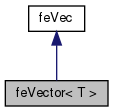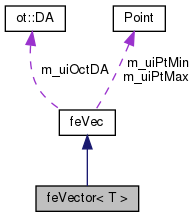Dendro  5.01 Dendro in Greek language means tree. The Dendro library is a large scale (262K cores on ORNL's Titan) distributed memory adaptive octree framework. The main goal of Dendro is to perform large scale multiphysics simulations efficeiently in mordern supercomputers. Dendro consists of efficient parallel data structures and algorithms to perform variational ( finite element) methods and finite difference mthods on 2:1 balanced arbitary adaptive octrees which enables the users to perform simulations raning from black holes (binary black hole mergers) to blood flow in human body, where applications ranging from relativity, astrophysics to biomedical engineering.
feVector< T > Class Template Referenceabstract

class that derived from abstract class feMat RHS computation of the weak formulation More...

`#include <feVector.h>`

Inheritance diagram for feVector< T >:[legend]
Collaboration diagram for feVector< T >:[legend]

## Public Member Functions

feVector (ot::DA *da, unsigned int dof=1)
constructs an FEM stiffness matrix class. More...

virtual void computeVec (const VECType *in, VECType *out, double scale=1.0)
Evaluates the RHS of the PDE at specific points (for example evaluation at the quadrature points) More...

virtual void elementalComputVec (const VECType *in, VECType *out, double *coords=NULL, double scale=1.0)=0
evalVec for the elemental vec More...

T & asLeaf ()

bool preComputeVec (const VECType *in, VECType *out, double scale=1.0)

bool postComputeVec (const VECType *in, VECType *out, double scale=1.0)

bool preEvalVec (const VECType *in, VECType *out, double scale=1.0)

bool postEvalVec (const VECType *in, VECType *out, double scale=1.0)Public Member Functions inherited from feVec
feVec (ot::DA *da)
: feVec constructor More...

~feVec ()
deconstructor

void setProblemDimensions (const Point &pt_min, const Point &pt_max)
set the problem dimension

virtual void setPlaceholder (const double *v)

## Protected Attributes

unsigned int m_uiDof
number of unknowns

VECType * m_uiEleVecIn
element nodal vec in

VECType * m_uiEleVecOut

double * m_uiEleCoordsProtected Attributes inherited from feVec
ot::DAm_uiOctDA
: pointer to OCT DA

ot::DAType m_uiDaType
: type of the DA

Point m_uiPtMin
problem domain min point

Point m_uiPtMax
problem domain max point

## Detailed Description

### template<typename T> class feVector< T >

class that derived from abstract class feMat RHS computation of the weak formulation

## ◆ feVector()

template<typename T >
 feVector< T >::feVector ( ot::DA * da, unsigned int dof = `1` )

constructs an FEM stiffness matrix class.

Parameters
 [in] da octree DA

## ◆ computeVec()

template<typename T >
 void feVector< T >::computeVec ( const VECType * in, VECType * out, double scale = `1.0` )
virtual

Evaluates the RHS of the PDE at specific points (for example evaluation at the quadrature points)

Parameters
 [out] out : function evaluated at specific points. Evaluates the right hand side of the weak formulations. Typically the mass matrix multiplied with the load function. [in] in Input vector (f) [out] out : Output vector (Mf)

Implements feVec.

## ◆ elementalComputVec()

template<typename T>
 virtual void feVector< T >::elementalComputVec ( const VECType * in, VECType * out, double * coords = `NULL`, double scale = `1.0` )
pure virtual

evalVec for the elemental vec

elemental compute vec which evaluate the elemental RHS of the weak formulation

Implemented in HeatEq::HeatVec.

## ◆ m_uiEleCoords

template<typename T>
 double* feVector< T >::m_uiEleCoords
protected

elemental coordinates

The documentation for this class was generated from the following file: